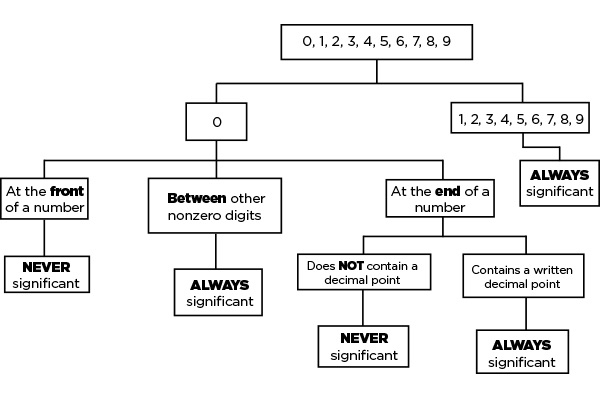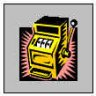Technical Article

# Significant Digits and Engineering Units

February 09, 2016 by Michael Stock

## Some rules of thumb for using significant digits and mitigating error propagation.

Learn the basics of using significant digits for mitigating error propagation.

When looking at a number, there are rules to determine which digits are significant and which aren't. As a byproduct of these rules, when the time arises to take measurements and perform several math operations to arrive at a resolution, it's important to consider these rules and to round appropriately in order to mitigate error propagation. Below is a list of rules one should follow in order to determine if a given digit is considered significant.

The following ARE significant digits:

• Zeros that are within a number (EX: 240503)
• Zeros that aren't used to hold a decimal point (EX:  98.600)
• All non-zero numbers (EX: 123456789)

The following are NOT significant digits:

• Zeros that set the decimal point (EX: 0.0025)

The following might be significant, depending on the situation:

• Zeros that follow a number (EX: 1200)

The last bullet can be applicable for both categories as it is a condition that is subjective for each case. It is common to round to an even 100, such as the example 1200, but the figure may be accurate to 4 places making 1200 contain 4 significant digits. Some people even underline the last significant figure in a number in order to mitigate ambiguity.### Reducing Error Propagation

When applying mathematics to significant figures, the answer will usually carry forward the least number of significant digits as any term from the problem. If I have 3 terms and 2 of them have 4 significant figures and the third has 2 significant figures, then the answer will normally only have 2 significant digits. The mathematical resolution is only as sound as the most uncertain term (AKA a chain is only as strong as its weakest link). There are some instances where simplification will not work, here are a few examples:

• 12345 + 23 = 12358 (it does NOT become 12000 just because one of the operands only has two significant figures).
• 823 + 491 = 1314 (the answer has more significant figures than either of the operands).

When terms can't be simplified, you must determine their uncertainty, which is a range of numbers that the true value lies between. It's good practice when taking measurements to always maximize the resolution of a given measurement, knowing that after calculations are complete there will be rounding that will reduce the precision of the measurements in question.

### Engineering Units

Engineering notation is a version of scientific notation that is written in powers of 10, where the exponents are multiples of 3. Every 3rd power of 10 has a prefix associated with it, for both greater and less than zero. The most common units are below:

• Tera  1012
• Giga  109
• Mega 106
• Kilo    103
• Milli    10-3
• Micro  10-6
• Nano  10-9
• Pico   10-12

The following statements are equivalent and each has the same number of significant digits:

This input can handle 20A.

This input can handle 20000mA.

This input can handle 0.02kA.

### Conclusion

The rules governing significant digits are designed to carry forward meaningful data, such that the mathematical resolution contains the least amount of uncertainty. Adhering to the rules is not only good practice but also the best method to mitigate error propagation.

BONUS: Since it's not a native format if you would like to use engineering notation in Excel, use the following custom format for the cells you wish to display:

###.###E+0• R
RUBEN_M3D1N4 February 11, 2016

Wouldn’t the last answers be from 20A to
0.02mA
20000kA
???

Like.
•tim yb February 12, 2016
Thanks for pointing that out! I accidentally put an extra zero in there when I formatted this piece. The engineering units are correct though.
Like.
•hp1729 February 26, 2016

The math is correct but we live in a world with resistors of 5% tolerance, capacitors of 20% tolerance ... three significant digits? Does it matter? An 8-bit ADC with 5 Volts full scale can’t distinguish smaller than 20 mV increments. On top of that we typically take 10 readings and average the results. Are precise calculations really necessary?
The LED is rated at 20 mA??? I drive it at 2 mA to 5 mA. I just don’t need full brightness and I often find it valuable to save current. I can do a lot with that 15 to 18 mA. Precise calculations for ballast resistors? Never.

Like.